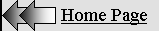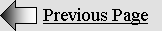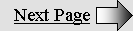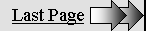ACTIVE  AND  REACTIVE  MASS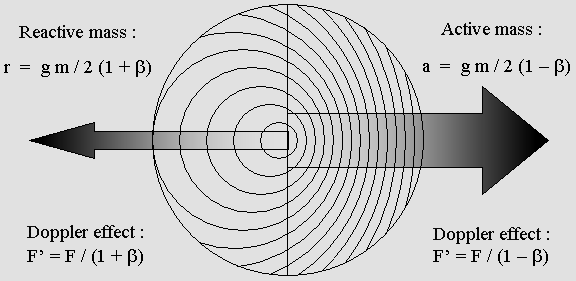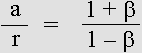Action and reaction forces are unequal because they are caused by waves undergoing the Doppler effect.

The Doppler effect in wavelength is  1 + beta  backward and   1 beta  forward.

The ratio is the same for action and reaction forces and for the Doppler effect.

The gamma factor = 1 / (1 beta 2) (1 / 2) can also be deduced from the Doppler effect: gamma = (a + r) / m

The mass gain according to: gamma * m m  is also given by:  a + r m in accordance with the Doppler effect.

This strongly suggests that matter is made of waves.

 Active and reactive mass. Hendrick A. Lorentz predicted that any fast moving material body should undergo a mass increase. Because matter is made of waves, which should undergo the Doppler effect, its mass or energy increase should be easily evaluated.   The mass must be divided into two parts: active and reactive mass. In order to explain action and reaction, the mass waves traveling forward will be considered active, while backward ones will be reactive. Let's take an example: suppose that a material body is moving at 86.6% of the speed of light. The Lorentz beta normalized speed is .866: beta  =  v / c The Lorentz transformation  g  value is .5: g = (1 – beta 2) 1 / 2 The gamma factor (the reciprocal 1 / g) is 2 : gamma  =  1 / (1 – beta 2) 1 / 2 So, according to Lorentz : beta = .866 ; g = .5 ; gamma = 2. In order to make things simple, the mass at rest is 1 kg, hence : m = 1. And because the gamma factor is 2, Lorentz predicts that the total mass M will be increased to 2 kg at 86.6% of the speed of light: M = gamma * m. Now, let's divide this total mass M into active and reactive parts. While at rest, they both equal .5 kg. However, because this wave system is moving at .866 c, it should also reduce its frequency according to Lorentz: F' = g F. This means that the wave energy should also be reduced according to g. In addition, those parts undergo the Doppler effect in opposite directions. The forward wavelength contraction is 1 – b and the backward dilation, 1 + b. Finally, the active and reactive mass values will be transformed in accordance with equations below:

 a = g m / 2 (1 – beta)               r = g m / 2 (1 + beta)

Active mass:   a = 1.866 kg      Reactive mass:  r = .134 kg

Total mass:  M = 1.866 + .134 = 2 kg

M = a + r

M = gamma * m

 The total mass M is indeed increased to 2 kg exactly as predicted by Lorentz, according to the gamma factor. Actually, the diagram below shows that the mass has been increased in accordance with the Doppler effect. The point is that the wavelength forward contraction is much more severe than the backward dilation. The contraction is unlimited. But the dilation never exceeds 2 times the original wavelength.Active and reactive mass, explaining action and reaction.

Here, the system speed is .5 c, half the speed of light.

 Note the equivalence: beta = (a – r) / (a + r) On the other hand, the wavelength ratio  R  is given by: R = (1 + beta) / (1 – beta) Or: R = a / r So the ratio is the same:a  is the active mass, whose contracted waves exert an active force forward.

r  is the reactive mass, whose dilated waves exert a reactive force backward.

The same ratio indicates that mass evolves the same way as the Doppler effect does.

 The values 1 + beta and 1 – beta are typical of the Doppler effect. They obviously indicate that such a mass increase is directly linked to the Doppler effect. I would like to emphasize here that Mr. Milo Wolff had already noticed this. He did not propose the correct formulas, though. One hundred years ago, Lorentz predicted such a mass increase, as well as clocks ticking slower. Today, those effects have been carefully verified. Because they are linked to the Doppler effect, this strongly indicates that matter is made of waves.

ACTIVE AND REACTIVE FORCES

 In addition, because waves are carrying energy, the mass indicates that matter contains energy, which implies active and reactive forces. Such forces are the result of the radiation pressure. They clearly involve waves. Let's repeat that while two observers are moving at the same speed and in the same direction, they cannot detect the Doppler effect between them. This explains why two electrons moving together will act and react as if they were at rest. Their behavior is relative. Newton established that action and reaction should be equal, but this is true only from their own point of view. Action and reaction forces always seem equal from those observers' own point of view. Henri Poincaré discovered in 1904 that the laws of all physical phenomena are the same whatever the system speed. He predicted that this should lead to some "new mechanics"; he was right. From an absolute point of view, though, all forces are subject to the Doppler effect. This should seem obvious in the animated diagrams below. It shows what is wrongly called "standing waves". These waves actually are moving to the right at .5 c, and you must imagine that you are following them at the same speed. Please observe that both the frequency and the amplitude are different forward and backward.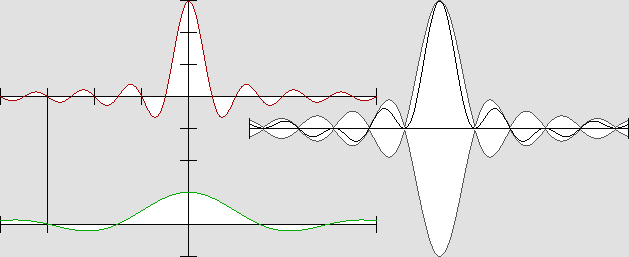"Pseudo-standing waves" in the vicinity of the electron's central core.

Active mass waves are moving to the right and reactive ones to the left.

The A amplitude (and wavelength)  R  ratio is given by: R = (1 + beta) / (1 beta)

Here, v = .5 c, hence: beta = .5 ;  R = 3;  A1 = 75 % ; A2 = 25 %.

 The diagram shown above represents the electron's active and reactive waves along the displacement axis only. Observe that the compressed waves are three times shorter, but that they also seem to move to the right three times slower. So their effective frequency (more exactly their rate) remains the same in both directions. This explains why the Doppler effect cannot be detected inside any moving frame of reference. For the same reason, any electron following or preceding another one with act or react with it in the same way. The animated diagram below shows plane "pseudo-standing waves":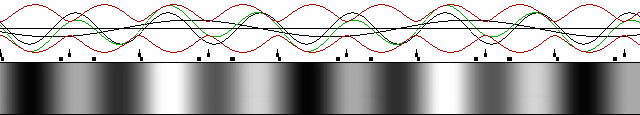Plane "pseudo-standing waves":  v = .5 c ;  beta = .5 ;  A1 = 65 % ;  A2 = 35 %.

Here, you must imagine that you are moving to the right at the same speed.

The nodes and antinodes are bouncing on each other as a result of opposite forces;

this explains the radiation pressure mechanism.

 The above diagram shows that the nodes' and antinodes' speed is not constant inside a pseudo-standing wave set while both the wavelength and the amplitude are not the same.  In addition to being pushed forward, they are somehow "shaken" as a consequence of the non linearity of the active and reactive forces. Radiation pressure. This phenomenon indicates that the electron central antinode should be very sensitive to the radiation pressure caused by any additional wave. While more waves are added from one direction, the electron normal inertia is destroyed. The particle can be accelerated or slowed down. It can also be deviated.   I made a very sophisticated computer program in order to show how ingoing hemispheric waves would behave in the vicinity of their center of curvature. This program does not use any equation, just Huygens' Principle. Because this principle has never been invalidated, this program is the most accurate and reliable ever. If you do not believe me, please note that this phenomenon can be verified using hemispheric ingoing sound waves.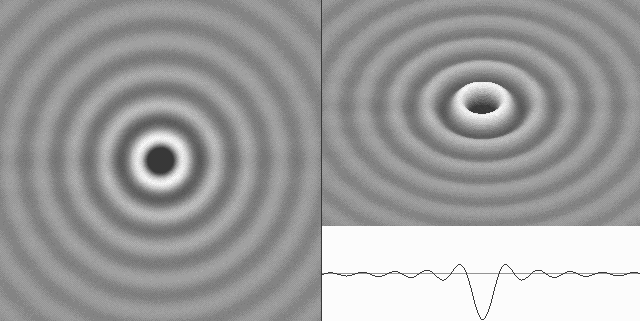Hemispheric waves incoming from the left and outgoing to the right, simulating the immobile electron's active mass.

The complete electron stays at rest because of the identical and opposite wave set, which contains the reactive mass.

But as soon as the active waves become stronger, the central antinode is constantly pushed forward.

 Any material body acts or reacts as if it were a finite box containing millions of rubber balls which where constantly moving in all directions.

The rubber balls analogy.

 In order to avoid losses, one can imagine an orbiting metal box containing 100% vacuum and hundreds of such moving rubber balls which are postulated to be absolutely lossless. If the box is accelerating, those balls become faster in the direction of motion. Conversely, they become slower backward. They undergo a sort of Doppler effect, but the wave contraction or dilation is reverted. The balls become more distant from each other in the direction of motion. This effect is cancelled by the balls speed, which is faster. When they are bouncing on the box surface, they communicate their kinetic energy to the box. Let's consider that the box itself has no mass and no inertia. This phenomenon may explain why such a moving box will go on moving. It will indeed explain inertia. As seen above, the active mass waves are pushing on the electron's core this way, explaining both its speed and inertia. Finally, lets suppose that a second box hits the first one. During this process there is no box surface between them. Then the balls will move freely from one box to another; they will push on the boxes opposite surface. This is the equivalent of the radiation pressure. Moreover a certain number of balls will definitely be transferred to the first box. This is the equivalent of the increase in mass. Basically and mechanically, the active and reactive mass work like this. But such a wonder can only be achieved by waves. Once again, this strongly indicates that matter is made of waves. The speed of light is an insuperable limit. The active mass, hence the total mass trends toward infinite while a material body approaches the speed of light. This means that Poincaré was amazingly right in 1904 when he stated that the speed of light is an insuperable limit. Many people misapprehend that some phenomena involve speeds faster then the speed of light, but they are wrong. On the one hand, matter can approach the speed of light, but it simply cannot reach it. On the other hand, all forces are transmitted by aether waves, whose speed is constant and equal to that of light. Firstly, action and reaction implies simultaneous effects, and this may induce an error. Secondly, one can also act on some invisible and unknown intermediate field such as an electromagnetic one. This field is made of plane standing waves between two electrons. It may be considered as virtual matter which is fully subject to the radiation pressure even when those electrons are very distant from each other. Clearly, while acting on this field, one will obtain a simultaneous effect on both electrons, but this effect will seem to have happened instantly.  Because photons do not exist, one cannot affirm that those photons may have changed suddenly and simultaneously well after the light has been emitted. One must realize that light is transmitted without any change only inside a vacuum. As soon as it encounters matter, which may be transparent as well, some new light is constantly created which interferes with the previous one. Its polarization or its phase may certainly be modified during the propagation process. So any instantaneous action at a distance is impossible. Some experiments may indicate that it is possible, but they surely have been misinterpreted.| 01 | 02 | 03 | 04 | 05 | 06 | 07 | 08 | 09 | 10 | 11 | 12 | 13 | 14 | 15 | You are here. |

| 17 | 18 | 19 | 20 | 21 | 22 | 23 | 24 | 25 | 26 | 27 | 28 | 29 | 30 | 31 | 32 | 33 |

 Gabriel LaFreniere Bois-des-Filion in Québec. On the Internet since September 2002. Last update December 3, 2009.